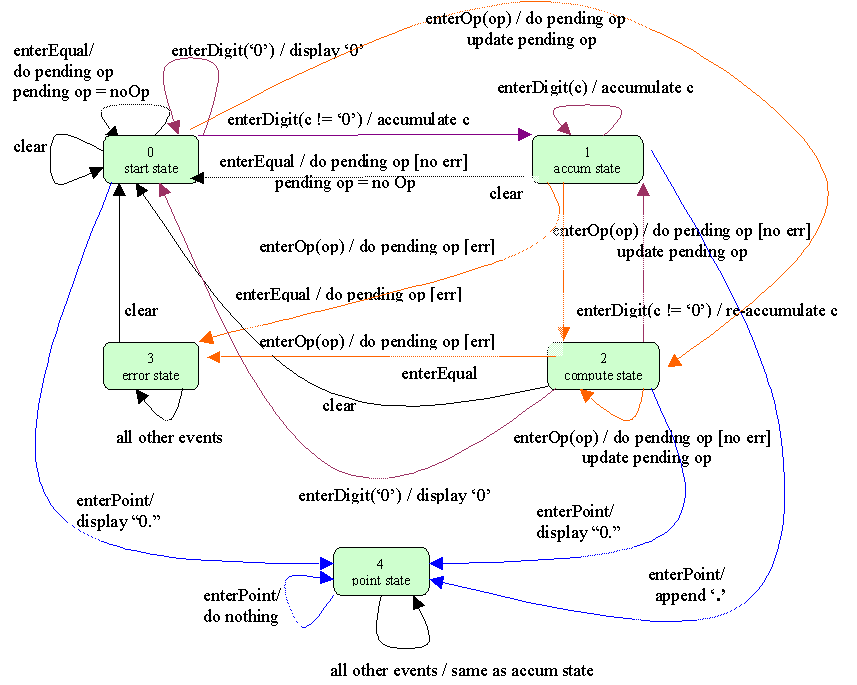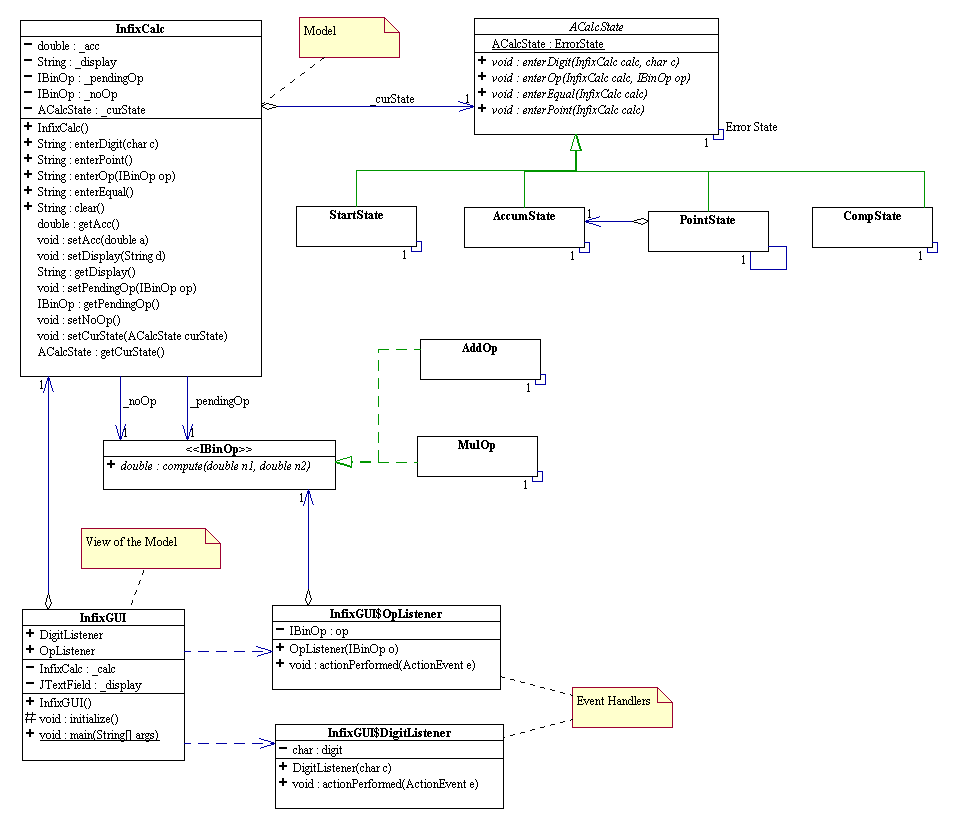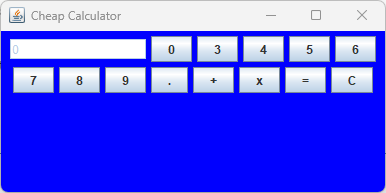# Finite State Machines and the State Design Pattern

(Back to Connexions Modules Home)

Summary: Make use of finite state machines and the state design pattern to create a Java "Cheap Calculator".

In this lab we will create the Graphical User Interface ("GUI") "Cheap Calculator" program which can do the four basic arithmetic operations: addition, subtraction, multiplication and division.

## Demo

To see where we are going, download and run the demo As you can see this is a really cheap calculator: it misses a couple of digit buttons and does not support subtraction nor division. Nevertheless, it seems to calculate arithmetic expression in infix notation correctly. What do we mean by an "infix" expression? A binary operation is said to be in infix notation if the operator (e.g. +) is in between the two operands. There are also "postfix" and "prefix" expressions. The following table shows an example of each form of notation.

### EXAMPLE 1: Prefix, postfix, and infix notation

 Adding two numbers x, y together Infix Notation x + y Prefix Notation + x y Postfix Notation x y +

## Background Information

I am interested in writing a GUI application that simulates the behavior of a "cheap" infix calculator. As the user interface, this calculator has on the outside:

• 10 buttons labeled 0, 1, 2, 3, 4, 5, 6, 7, 8, 9, respectively, to enter digit characters
• one button labeled . to enter the decimal point
• one button labeled +, to perform addition
• one button labeled *, to perform multiplication
• one button labeled =, to compute the result of the input so far
• one button labeled C, to clear all entries in the calculator and reset the calculator to its pristine initial state.
• and a display text field for me to view the input and the result of my desired computation.

Inside this calculator is perhaps some kind of electronic circuit board capable of carrying out the actual computations. The GUI provides the only way for me to interact with the internals of the calculator. How do I want the calculator to behave? For a start, as I click on the digit buttons, I expect the calculator to accumulate the digits by concatenating them together and displaying the result on the display text field. But wait! What if I only click the digit '0' button? The calculator should not concatenate one '0' after another, should it? Run the demo and check it out yourself!

Now, try this: click '3', then click '+', then click '0' four times. This time around, clicking '0' results consistently in concatenating '0' to the existing display text. The calculator has changed its "click '0'" behavior after I click a digit button that is not a '0'. We say that the calculator has gone through a "state change" and behaves differently in each state.

Here is another example.

• First click C to reset the calculator. The display should show '0'.
• Now, click '3', then '3' again, then '3' again.
• What do you see? The first time you click '3', the calculator replaces '0' with '3'. Then for each subsequent click on '3', the calculator simply appends '3' to the current display text field and update it with the resulting string. You should see "333" at this point.
• Next, click '+': "333" is still being display. But now click '3'. What happens? '3' is no longer appended to the existing display string, isn't it? You are sending the same message to the calculator by clicking the same '3', but the calculator responds differently this time, doesn't it? It has dynamically changed its behavior after you clicked on '+'! Again what we see here is another example of "state change". Clicking the '+' causes the calculator to transition to another state.

The behaviors of my calculator can be modeled as what is called a "finite state machine." What is a state? How many states are there? How do we go about describing the behavior of the calculator as it changes from state to state?

## Finite State Machine (an informal discussion)

When you click on a button of the calculator, you are in effect making a request to the calculator to perform certain task. At any point in time, the internals of the calculator are in some specific conditions with specific values that would cause the calculator to respond in a very specific manner to such a request. The condition in which the calculator is in at any point in time is called the state of the calculator at that point in time. A state of a system is defined by the behaviors of the system. The user can interact with the calculator in only five ways:

• click a digit button,
• click an operation button,
• click the equal button,
• click the clear button,
• click the point button.

To specify the behavior of the calculator, we must specify the calculator's response to each of the above five clicking events.

### NOTE:

Reference the "state transition diagram" below for the following discussion.

### Start State

When you first turn on the calculator, imagine that it is in some initial condition called a start state. Suppose, from the start state, you...

• click a digit button: what do you want to happen? It depends...
• digit = '0': display '0' and not concatenating '0' on subsequent clicks on '0'. This implies the calculator can stay in the same start state when '0' is clicked.
• digit != '0': display the digit and begin concatenating any digit (including '0') on subsequent digit clicks. This implies the existence of another state for the calculator to switch to after a non-zero digit is clicked for the first time. Let's call this new state the accumulate state. We shall analyze the behavior of this state later.
• click an operation button, for instance, "+", which we will refer to as "op" for generalities sake. Among the many things we want to happens here, one thing for sure we want is for the calculator to remember op as a pending operation so that we can enter another number, click the equal button and get the correct result. A subtle problem arises here. Is there any current pending operation to be perform before updating op as the new pending operation? You may say, well, if we just turn on the calculator and the first thing you do is click an op button, then there is no pending operation to perform. The response to that is the calculator may transition form one state to another during its usage and may come back to the start state with some pending operation yet to be carried out. In any case, the calculator must have a way to remember a pending op, it is best to have the calculator remember a well-defined pending op at all times, even if there is no pending op at all. This is where the concept of a no-op operation becomes useful. Define the no-op operation to be an operation that "does nothing" and use no-op as a pending operation when there is no operation to perform. In the design pattern language, this is called the "Null Object" pattern. The next question to ask is whether or not the calculator should change to a state different from both the start state and the accumulate state.
• Can the calculator stay in the same start state? Say, in the beginning you start with the number 0 in the display, and you click '+' . This is tantamount to entering the expression 0 +. If you click the equal button next, then you are in essence asking the calculator to compute 0 +, which is an invalid infix expression. What do you want the calculator to do and display here? Examine a few real calculators of different brands and see how they behave. Also, examine a few different "software" calculators, if you have any. They do not all behave the same way, do they? The behavior here can be arbitrarily defined because the expression entered ( 0 + ) is not a valid infix expression. So we arbitrarily specify that our calculator display an error message, stop accepting any additional inputs and stop performing any additional operations. This implies the existence of a new state called the error state. With this new behavior specification, the calculator cannot stay in the same state as the start state because, as we shall see in the discussion of the click equal event that follows, the start state cannot display an error.
• Can the new state be the same as the accumulate state? If we transition to the accumulate state after we click an op, then we should be accumulating '0' as we continually click on '0', shouldn't we? But is that want we want the calculator to behave like? Of course not. After we click an op button, we should be in a state where we can accept new digit inputs. If we click '0', we do not want to accumulate '0' at all. Since this new state does not have the same behavior as the accumulate state discovered in 1, it is a different state. Let's call this state the compute state. We get to a compute state after we click on an op button.
• click the equal button: we want the calculator to compute the pending operation, which may be a no-op, update the pending operation to a no-op (since there is no more operation to perform), display the result and be ready to accept a brand new input. There is no need to transition to any state.
• click the clear button: we want the calculator to be reset to the initial pristine condition as if it has just been turned on. Obviously, the calculator should stay in this same start state.
• click the point button: we want to display "0." to signify that we have just entered a decimal point in order to input a number less than one. Do we stay in the same start state? We can't, because if we click '0' next, we want to see "0.". Should we stay in the start state, we would be displaying "0" instead. The new state is obviously not the error state either. Let's call this new state the point state.
• Can the point state be the compute state? Consider the following sequence of 4 clicks: '0', '.' , '0', '0'. After the first click, '0', the calculator stays in the same start state. If after the second click ('.'), we do not change state, then after the third click ('0'), and fourth click ('0'), the calculator would display "0" and not "0.00", as it should. Therefore the point state cannot be the compute state.
• Can the point state be the accumulate state? It depends on what we want the calculator to behave like once we are in the point state and keep clicking '.'. The first '.' click will cause the calculator to display "0.". What do we want the computer to display if click '.' again? One reasonable behavior is to ignore the '.' click and do nothing. That is, once we are in the point state, we ignore all the point clicks. What should the accumulate state behave like when we click '.'? Can we ignore the point click once we are in the accumulate state? From the start state, we click '3' and change to the accumulate state as discussed in the above. Now, click '.'. If calculator ignores the point click and does nothing, then we cannot enter the decimal point at all. We can never enter something like "3.5". The accumulate state cannot ignore the first point click while the point state ignores all point clicks. Thus the point state and the accumulate state are not the same.

Beginning with one state, the start state, by analyzing and specifying the behavior of the calculator in response to the five distinct click events, we have inferred the existence of four distinct other states: accumulate state, compute state, point state and error state. We can repeat the same analysis on each of these states for each of the click events. We will not discussed them in details here. Instead, we summarize the state behaviors and the state transition in the form of a diagram shown below.

Figure 1: State Transitions
State Diagram## State Design Pattern

Traditional procedural programming implements the behavior of finite state machines by using numbers, loops and conditional constructs such as if and switch statements. In OOP, each state is an object with behaviors. A finite state machine is an object that has states. The machine holds a reference to an abstract state and delegates all state-dependent behaviors to its current state. This is the gist of what is called the state design pattern. We will apply the state pattern to model the cheap calculator. Here is the UML diagram of the complete system that we will be building:You may download the partially completed GUI code here: AFrame.java & InfixGUI.java (put them in a package called "infixView")

### Let's get started! Remember to do the following parts in order:

Warning! As you progress through the lab, the directions will deliberately get less and less detailed, as you are expected to figure more and more of the process out on your own!

## Step 0: Understand the problem

Study the above state transition diagram!

## Step 1: Calculator with no Concrete States

• Create stub class files for InfixCalc, ACalcState, IBinOp, AddOp and MulOp as shown in the UML class diagram; be sure to put it in the infixModel subdirectory (package).
• Make AddOp and MulOp singletons and write the code for their compute() methods. This should be trivial.
• Look at the javadoc/UML documentation of the ErrorState field in ACalcState. It is set to a "do-nothing" state. This is called the "Null Object Pattern". Copy the code for ErrorState to your ACalcState file.
• Add appropriate System.out.println statements to the public methods of InfixCalc, AddOp and MulOp.
• Compile and run; click all the buttons to prove that your listeners are really listening.

## Step 2: Add Concrete States

• Create concrete classes StartState, AccumState, PointState and CompState as shown in the javadoc/UML diagram.
• Be sure to make them singletons.
• Add appropriate System.out.println statements to their public methods.
• Now, go back to InfixCalc and apply the state pattern by delegating all state-dependent behaviors to the state. Is clear() a state-dependent behavior?
• Compile and run to see that the delegations are made.
• Use the above state diagram to write the code for all the methods of each of the concrete state classes, one class at a time.
• Compile and manually test each of the classes by testing each of the click events.

## Step 3: Add More Buttons and Operations

• Modify InFixGUI to add a '1' button and a '2' button. Add appropriate event listeners for these two buttons.
• Add a subtraction binary operation and a division binary operation.
• Modify InFixGUI to add a subtraction button and a division button. Add appropriate event listeners for these two buttons.
• Compile and run and test at each of the above steps.
• You now should have a fully functional "cheap" calculator.

## Calculator demoOriginally published in Connexions (CNX): https://web.archive.org/web/20110415122613/http://cnx.org/content/m17257/latest/